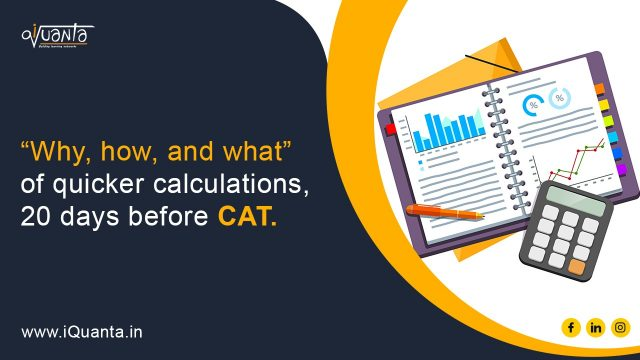Take a look at the picture below, can you identify who this is?She is Shakuntala Devi, who was known as the “Human Computer”. She was able to calculate the 23rd root of a 201-digit number:

916748676920039158098660927585380162483106680144308622407126516427934657040867096593279205767480806790022783016354924852380335745316935111903596577547340075681688305 620821016129132845564805780158806771

in 50 seconds in 1977, a feat that the computers at that time took 80 seconds to do. The speed at which she was able to do such calculations was something that made her famous around the world!

Now as an MBA aspirant, you do not need to be a Shakuntala Devi in your entrance exams, but you do need to learn the art of speeding up your calculations. A question may be there in your mind, how is this going to help me? Why should I put effort in improving my calculation speed? If I need to improve it, how do I go about it? Let us look at them one by one:

1. Why: Why do I need to focus on improving my calculation speed?

Let us look at an exam like CAT. As per the new format, the time per section is 40 mins and you have 20-24 question in the section.

So, per question you have 40/20= 2 mins or 40/24=1 min 40 secs. Now this is the best-case scenario for you, but we know that it might not be possible for all.Say you want to attempt 80% of the questions, those you are sure of. That is 16 (for 20 Q) or 19(for 24Q). You are looking at 2 mins 30 sec or 2 mins per question.

Now if you take time for calculations, for each question you may overshoot the time limit. Say for 16 questions you are just taking 1 min more for calculations per question. That means 3 min 30 sec per question. The MAX you will be able to attempt is just 10-11 questions (out of the 16) and this number will keep on reducing as you take more time for calculations per question. So being good at calculations is not an option (this applies to DILR too).2. How: How can I improve on this?

Now we get to the main part. How can you get around getting better at calculations? Here are a few tips for that:• Multiplication tables, squares, cubes, and square roots are your friends

Now this may sound like something you will tell a child in school, but this is way more important than you think. Knowing the number operations (multiplication tables, squares, roots, cubes) for 1-25 are essential as if you have these at your fingertips, you will not spend the 30 secs calculating these mechanical operations. This applies not only for the CAT but all entrances you can think of.

The link of 1/x and percentages is really important. If you need to know what % is 2/13, if you have 1/13 memorized it is just a matter of doubling it, else you will sit and calculate it. This will be really useful in DILR where 1/x and percentages frequently pop up. If you know these beforehand, you do not have to think about calculating these things and sweep through the problems. For this you can memorize 1/1 to 1/25 and their % equivalent.

• Practice Practice Practice!

Now you practice VARC, DILR and quants, but you also need to practice your calculations. Just knowing the numbers will not be enough. Make sure you use some resources to aid you with this. You can use the speed math resources you have or even websites like https://www.mathtrainer.org/ that will help you speed up calculations.